Courses

# Logic Gates MCQ

## 10 Questions MCQ Test Topic wise Tests for IIT JAM Physics | Logic Gates MCQ

Description
This mock test of Logic Gates MCQ for Physics helps you for every Physics entrance exam. This contains 10 Multiple Choice Questions for Physics Logic Gates MCQ (mcq) to study with solutions a complete question bank. The solved questions answers in this Logic Gates MCQ quiz give you a good mix of easy questions and tough questions. Physics students definitely take this Logic Gates MCQ exercise for a better result in the exam. You can find other Logic Gates MCQ extra questions, long questions & short questions for Physics on EduRev as well by searching above.
QUESTION: 1

### The output of an AND gate with 3 inputs A, B and C is high when :

Solution:

The correct answer is :A = 1 , B = 1 , C = 1

QUESTION: 2

Solution:

QUESTION: 3

### What is the expression for a 4 input OR gate?

Solution:

The correct answer is: Y= A + B + C + D

QUESTION: 4

If a signal passing through a gate is inhibited by sending a LOW into one of the inputs and the output is HIGH, the gate is a(n)

Solution:

QUESTION: 5

It is required to change a logic 1 to logic 0 and vice versa the logic gate required to do this is :

Solution:

QUESTION: 6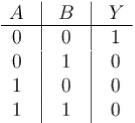What gate corresponds to the above logic gate?

Solution:

The correct answer is: NOR gate

QUESTION: 7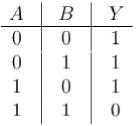What gate corresponds to the logic gate shown above :

Solution:

QUESTION: 8

Outputs from an AND gate and OR gate are fed into a 2 input NAND gate the output is :

Solution: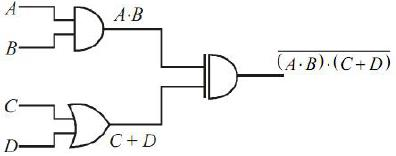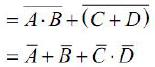The correct answer is: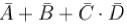QUESTION: 9

Which of the following is true fo r an exclusive OR gate?

Solution:

The correct answer is: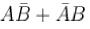QUESTION: 10

If a 3 input NOR gate has eight input possibilities how many of them will result in a high output?

Solution: